# Package MKpower

## Introduction

The package includes functions for power analysis and sample size calculation for Welch and Hsu (Hedderich and Sachs 2018) t tests including Monte-Carlo simulations of empirical power and type-I-error. In addition, power and sample size calculation for Wilcoxon rank sum and signed rank tests via Monte-Carlo simulations can be performed. Moreover, power and sample size required for the evaluation of a diagnostic test(-system) (Flahault, Cadilhac, and Thomas 2005; Dobbin and Simon 2007) as well as for a single proportion (Fleiss, Levin, and Paik 2003), comparing two negative binomial rates (Zhu and Lakkis 2014), and ANCOVA (Shieh 2020) can be calculated.

library(MKpower)

## Single Proportion

The computation of the required sample size for investigating a single proportion can be determined via the respective test or confidence interval (Fleiss, Levin, and Paik 2003). First, we consider the asymptotic test.

## with continuity correction
power.prop1.test(p1 = 0.4, power = 0.8)
##
##      Power calculation for testing a given proportion (with continuity correction)
##
##               n = 203.7246
##           delta = 0.1
##              p1 = 0.4
##              p0 = 0.5
##       sig.level = 0.05
## exact.sig.level = 0.04205139
##           power = 0.8
##     exact.power = 0.8008049
##     alternative = two.sided
##
## NOTE: n = total sample size
## without continuity correction
power.prop1.test(p1 = 0.4, power = 0.8, cont.corr = FALSE)
##
##      Power calculation for testing a given proportion
##
##               n = 193.8473
##           delta = 0.1
##              p1 = 0.4
##              p0 = 0.5
##       sig.level = 0.05
## exact.sig.level = 0.05228312
##           power = 0.8
##     exact.power = 0.8067065
##     alternative = two.sided
##
## NOTE: n = total sample size

Next, we compute the sample size via the respective asymptotic confidence interval.

## with continuity correction
ssize.propCI(prop = 0.4, width = 0.14)
##
##      Sample size calculation by method of wald-cc
##
##               n = 202.1865
##            prop = 0.4
##           width = 0.14
##      conf.level = 0.95
##
## NOTE: Two-sided confidence interval
## without continuity correction
ssize.propCI(prop = 0.4, width = 0.14, method = "wald")
##
##      Sample size calculation by method of wald
##
##               n = 188.1531
##            prop = 0.4
##           width = 0.14
##      conf.level = 0.95
##
## NOTE: Two-sided confidence interval

## Welch Two-Sample t-Test

For computing the sample size of the Welch t-test, we only consider the situation of equal group size (balanced design).

## identical results as power.t.test, since sd = sd1 = sd2 = 1
power.welch.t.test(n = 20, delta = 1)
##
##      Two-sample Welch t test power calculation
##
##               n = 20
##           delta = 1
##             sd1 = 1
##             sd2 = 1
##       sig.level = 0.05
##           power = 0.8689528
##     alternative = two.sided
##
## NOTE: n is number in *each* group
power.welch.t.test(power = .90, delta = 1)
##
##      Two-sample Welch t test power calculation
##
##               n = 22.0211
##           delta = 1
##             sd1 = 1
##             sd2 = 1
##       sig.level = 0.05
##           power = 0.9
##     alternative = two.sided
##
## NOTE: n is number in *each* group
power.welch.t.test(power = .90, delta = 1, alternative = "one.sided")
##
##      Two-sample Welch t test power calculation
##
##               n = 17.84713
##           delta = 1
##             sd1 = 1
##             sd2 = 1
##       sig.level = 0.05
##           power = 0.9
##     alternative = one.sided
##
## NOTE: n is number in *each* group
## sd1 = 0.5, sd2 = 1
power.welch.t.test(delta = 1, sd1 = 0.5, sd2 = 1, power = 0.9)
##
##      Two-sample Welch t test power calculation
##
##               n = 14.53583
##           delta = 1
##             sd1 = 0.5
##             sd2 = 1
##       sig.level = 0.05
##           power = 0.9
##     alternative = two.sided
##
## NOTE: n is number in *each* group

## Hsu Two-Sample t-Test

For computing the sample size of the Hsu t-test (Hedderich and Sachs 2018), we only consider the situation of equal group size (balanced design).

## slightly more conservative than Welch t-test
power.hsu.t.test(n = 20, delta = 1)
##
##      Two-sample Hsu t test power calculation
##
##               n = 20
##           delta = 1
##             sd1 = 1
##             sd2 = 1
##       sig.level = 0.05
##           power = 0.8506046
##     alternative = two.sided
##
## NOTE: n is number in *each* group
power.hsu.t.test(power = .90, delta = 1)
##
##      Two-sample Hsu t test power calculation
##
##               n = 23.02186
##           delta = 1
##             sd1 = 1
##             sd2 = 1
##       sig.level = 0.05
##           power = 0.9
##     alternative = two.sided
##
## NOTE: n is number in *each* group
power.hsu.t.test(power = .90, delta = 1, alternative = "one.sided")
##
##      Two-sample Hsu t test power calculation
##
##               n = 18.5674
##           delta = 1
##             sd1 = 1
##             sd2 = 1
##       sig.level = 0.05
##           power = 0.9
##     alternative = one.sided
##
## NOTE: n is number in *each* group
## sd1 = 0.5, sd2 = 1
power.welch.t.test(delta = 0.5, sd1 = 0.5, sd2 = 1, power = 0.9)
##
##      Two-sample Welch t test power calculation
##
##               n = 53.86822
##           delta = 0.5
##             sd1 = 0.5
##             sd2 = 1
##       sig.level = 0.05
##           power = 0.9
##     alternative = two.sided
##
## NOTE: n is number in *each* group
power.hsu.t.test(delta = 0.5, sd1 = 0.5, sd2 = 1, power = 0.9)
##
##      Two-sample Hsu t test power calculation
##
##               n = 54.49421
##           delta = 0.5
##             sd1 = 0.5
##             sd2 = 1
##       sig.level = 0.05
##           power = 0.9
##     alternative = two.sided
##
## NOTE: n is number in *each* group

## ANCOVA

With function power.ancova one can compute power and sample size in ANCOVA designs (Shieh 2020). The default matrix of contrasts used in the function is

## 3 groups
cbind(rep(1,2), -diag(2))
##      [,1] [,2] [,3]
## [1,]    1   -1    0
## [2,]    1    0   -1
## 4 groups
cbind(rep(1,3), -diag(3))
##      [,1] [,2] [,3] [,4]
## [1,]    1   -1    0    0
## [2,]    1    0   -1    0
## [3,]    1    0    0   -1

We use the example provided in Table 9.7 of Maxwell and Delaney (2004).

## Example from Maxwell and Delaney (2004) according to Shieh (2020)
power.ancova(mu=c(7.5366, 11.9849, 13.9785), var = 29.0898, power = 0.8)
##
##      ANCOVA power calculation
##
##              ns = 14.29481, 14.29481, 14.29481
##              mu = 7.5366, 11.9849, 13.9785
##             var = 29.0898
##         nr.covs = 1
##       sig.level = 0.05
##           power = 0.8
##
## NOTE: Total sample size: 45
power.ancova(n = rep(45/3, 3), mu=c(7.5366, 11.9849, 13.9785), var = 29.0898)
##
##      ANCOVA power calculation
##
##              ns = 15, 15, 15
##              mu = 7.5366, 11.9849, 13.9785
##             var = 29.0898
##         nr.covs = 1
##       sig.level = 0.05
##           power = 0.8219894
##
## NOTE: Total sample size: 45
power.ancova(mu=c(7.5366, 11.9849, 13.9785), var = 29.0898, power = 0.9)
##
##      ANCOVA power calculation
##
##              ns = 18.32456, 18.32456, 18.32456
##              mu = 7.5366, 11.9849, 13.9785
##             var = 29.0898
##         nr.covs = 1
##       sig.level = 0.05
##           power = 0.9
##
## NOTE: Total sample size: 57
power.ancova(n = rep(57/3, 3), mu=c(7.5366, 11.9849, 13.9785), var = 29.0898)
##
##      ANCOVA power calculation
##
##              ns = 19, 19, 19
##              mu = 7.5366, 11.9849, 13.9785
##             var = 29.0898
##         nr.covs = 1
##       sig.level = 0.05
##           power = 0.9115114
##
## NOTE: Total sample size: 57

Based on the reported adjusted group means and error variance, a (total) sample size of 45 is required to achieve a power of at least 80%. The calculated power is 82.2%. With a sample size of 57 the power will be at least 90%, where the calculated power is 91.2%.

Introducing additional covariates (random covariate model) will increase the required sample size.

power.ancova(mu=c(7.5366, 11.9849, 13.9785), var = 29.0898, power = 0.8,
nr.covs = 2)
##
##      ANCOVA power calculation
##
##              ns = 14.65509, 14.65509, 14.65509
##              mu = 7.5366, 11.9849, 13.9785
##             var = 29.0898
##         nr.covs = 2
##       sig.level = 0.05
##           power = 0.8
##
## NOTE: Total sample size: 45
power.ancova(mu=c(7.5366, 11.9849, 13.9785), var = 29.0898, power = 0.8,
nr.covs = 3)
##
##      ANCOVA power calculation
##
##              ns = 15.01403, 15.01403, 15.01403
##              mu = 7.5366, 11.9849, 13.9785
##             var = 29.0898
##         nr.covs = 3
##       sig.level = 0.05
##           power = 0.8
##
## NOTE: Total sample size: 48
power.ancova(mu=c(7.5366, 11.9849, 13.9785), var = 29.0898, power = 0.8,
nr.covs = 5)
##
##      ANCOVA power calculation
##
##              ns = 15.72837, 15.72837, 15.72837
##              mu = 7.5366, 11.9849, 13.9785
##             var = 29.0898
##         nr.covs = 5
##       sig.level = 0.05
##           power = 0.8
##
## NOTE: Total sample size: 48
power.ancova(mu=c(7.5366, 11.9849, 13.9785), var = 29.0898, power = 0.8,
nr.covs = 10)
##
##      ANCOVA power calculation
##
##              ns = 17.4965, 17.4965, 17.4965
##              mu = 7.5366, 11.9849, 13.9785
##             var = 29.0898
##         nr.covs = 10
##       sig.level = 0.05
##           power = 0.8
##
## NOTE: Total sample size: 54

We can also calculate the per group sample sizes for an imbalanced design.

power.ancova(mu=c(7.5366, 11.9849, 13.9785), var = 29.0898, power = 0.8,
group.ratio = c(1, 1.25, 1.5))
##
##      ANCOVA power calculation
##
##              ns = 12.25390, 15.31738, 18.38085
##              mu = 7.5366, 11.9849, 13.9785
##             var = 29.0898
##         nr.covs = 1
##       sig.level = 0.05
##           power = 0.8
##
## NOTE: Total sample size: 48
power.ancova(n = c(13, 16, 19), mu=c(7.5366, 11.9849, 13.9785), var = 29.0898,
group.ratio = c(1, 1.25, 1.5))
##
##      ANCOVA power calculation
##
##              ns = 13, 16, 19
##              mu = 7.5366, 11.9849, 13.9785
##             var = 29.0898
##         nr.covs = 1
##       sig.level = 0.05
##           power = 0.8266307
##
## NOTE: Total sample size: 48
power.ancova(mu=c(7.5366, 11.9849, 13.9785), var = 29.0898, power = 0.8,
group.ratio = c(1, 0.8, 2/3))
##
##      ANCOVA power calculation
##
##              ns = 16.87449, 13.49959, 11.24966
##              mu = 7.5366, 11.9849, 13.9785
##             var = 29.0898
##         nr.covs = 1
##       sig.level = 0.05
##           power = 0.8
##
## NOTE: Total sample size: 43
power.ancova(n = c(17, 14, 12), mu=c(7.5366, 11.9849, 13.9785), var = 29.0898,
group.ratio = c(1, 0.8, 2/3))
##
##      ANCOVA power calculation
##
##              ns = 17, 14, 12
##              mu = 7.5366, 11.9849, 13.9785
##             var = 29.0898
##         nr.covs = 1
##       sig.level = 0.05
##           power = 0.8034654
##
## NOTE: Total sample size: 43

We get a smaller total sample size with an imbalanced design (43 instead of 45).

## Wilcoxon Rank Sum and Signed Rank Tests

For computing the sample size of these tests, we offer a function that performs Monte-Carlo simulations to determine their (empirical) power.

rx <- function(n) rnorm(n, mean = 0, sd = 1)
ry <- function(n) rnorm(n, mean = 0.5, sd = 1)
## two-sample
sim.ssize.wilcox.test(rx = rx, ry = ry, n.max = 100, iter = 1000)
##
##      Wilcoxon rank sum test
##
##               n = 10, 20, 30, 40, 50, 60, 70
##              rx = rnorm(n, mean = 0, sd = 1)
##              ry = rnorm(n, mean = 0.5, sd = 1)
##       sig.level = 0.05
##       emp.power = 0.163, 0.315, 0.444, 0.595, 0.672, 0.761, 0.831
##     alternative = two.sided
##
## NOTE: n is number in *each* group
sim.ssize.wilcox.test(rx = rx, ry = ry, n.min = 65, n.max = 70,
step.size = 1, iter = 1000, BREAK = FALSE)
##
##      Wilcoxon rank sum test
##
##               n = 65, 66, 67, 68, 69, 70
##              rx = rnorm(n, mean = 0, sd = 1)
##              ry = rnorm(n, mean = 0.5, sd = 1)
##       sig.level = 0.05
##       emp.power = 0.804, 0.795, 0.805, 0.811, 0.796, 0.812
##     alternative = two.sided
##
## NOTE: n is number in *each* group
power.t.test(delta = 0.5, power = 0.8)
##
##      Two-sample t test power calculation
##
##               n = 63.76576
##           delta = 0.5
##              sd = 1
##       sig.level = 0.05
##           power = 0.8
##     alternative = two.sided
##
## NOTE: n is number in *each* group
## one-sample
sim.ssize.wilcox.test(rx = ry, n.max = 100, iter = 1000, type = "one.sample")
##
##      Wilcoxon signed rank test
##
##               n = 10, 20, 30, 40
##              rx = rnorm(n, mean = 0.5, sd = 1)
##       sig.level = 0.05
##       emp.power = 0.280, 0.545, 0.761, 0.858
##     alternative = two.sided
##
## NOTE: n is number in *each* group
sim.ssize.wilcox.test(rx = ry, type = "one.sample", n.min = 33,
n.max = 38, step.size = 1, iter = 1000, BREAK = FALSE)
##
##      Wilcoxon signed rank test
##
##               n = 33, 34, 35, 36, 37, 38
##              rx = rnorm(n, mean = 0.5, sd = 1)
##       sig.level = 0.05
##       emp.power = 0.781, 0.791, 0.823, 0.837, 0.824, 0.854
##     alternative = two.sided
##
## NOTE: n is number in *each* group
power.t.test(delta = 0.5, power = 0.8, type = "one.sample")
##
##      One-sample t test power calculation
##
##               n = 33.3672
##           delta = 0.5
##              sd = 1
##       sig.level = 0.05
##           power = 0.8
##     alternative = two.sided
## two-sample
rx <- function(n) rgamma(n, scale = 2, shape = 1.5)
ry <- function(n) rgamma(n, scale = 4, shape = 1.5) # equal shape
## two-sample
sim.ssize.wilcox.test(rx = rx, ry = ry, n.max = 100, iter = 1000)
##
##      Wilcoxon rank sum test
##
##               n = 10, 20, 30
##              rx = rgamma(n, scale = 2, shape = 1.5)
##              ry = rgamma(n, scale = 4, shape = 1.5)
##       sig.level = 0.05
##       emp.power = 0.334, 0.644, 0.815
##     alternative = two.sided
##
## NOTE: n is number in *each* group
sim.ssize.wilcox.test(rx = rx, ry = ry, n.min = 25, n.max = 30,
step.size = 1, iter = 1000, BREAK = FALSE)
##
##      Wilcoxon rank sum test
##
##               n = 25, 26, 27, 28, 29, 30
##              rx = rgamma(n, scale = 2, shape = 1.5)
##              ry = rgamma(n, scale = 4, shape = 1.5)
##       sig.level = 0.05
##       emp.power = 0.761, 0.737, 0.777, 0.795, 0.806, 0.812
##     alternative = two.sided
##
## NOTE: n is number in *each* group

For practical applications we recommend to use a higher number of iterations. For more detailed examples we refer to the help page of the function.

## Two Negative Binomial Rates

When we consider two negative binomial rates (Zhu and Lakkis 2014), we can compute sample size or power applying function power.nb.test.

## examples from Table III in Zhu and Lakkis (2014)
power.nb.test(mu0 = 5.0, RR = 2.0, theta = 1/0.5, duration = 1, power = 0.8, approach = 1)
##
##      Power calculation for comparing two negative binomial rates
##
##               n = 22.37386
##              n1 = 22.37386
##             mu0 = 5
##              RR = 2
##           theta = 2
##        duration = 1
##       sig.level = 0.05
##           power = 0.8
##     alternative = two.sided
##
## NOTE: n = sample size of control group, n1 = sample size of treatment group
power.nb.test(mu0 = 5.0, RR = 2.0, theta = 1/0.5, duration = 1, power = 0.8, approach = 2)
##
##      Power calculation for comparing two negative binomial rates
##
##               n = 21.23734
##              n1 = 21.23734
##             mu0 = 5
##              RR = 2
##           theta = 2
##        duration = 1
##       sig.level = 0.05
##           power = 0.8
##     alternative = two.sided
##
## NOTE: n = sample size of control group, n1 = sample size of treatment group
power.nb.test(mu0 = 5.0, RR = 2.0, theta = 1/0.5, duration = 1, power = 0.8, approach = 3)
##
##      Power calculation for comparing two negative binomial rates
##
##               n = 20.85564
##              n1 = 20.85564
##             mu0 = 5
##              RR = 2
##           theta = 2
##        duration = 1
##       sig.level = 0.05
##           power = 0.8
##     alternative = two.sided
##
## NOTE: n = sample size of control group, n1 = sample size of treatment group

## Diagnostic Test

Given an expected sensitivity and specificity we can compute sample size, power, delta or significance level of diagnostic test (Flahault, Cadilhac, and Thomas 2005).

## see n2 on page 1202 of Chu and Cole (2007)
power.diagnostic.test(sens = 0.99, delta = 0.14, power = 0.95) # 40
##
##      Diagnostic test exact power calculation
##
##            sens = 0.99
##               n = 40
##              n1 = 40
##           delta = 0.14
##       sig.level = 0.05
##           power = 0.95
##            prev = NULL
##
## NOTE: n is number of cases, n1 is number of controls
power.diagnostic.test(sens = 0.99, delta = 0.13, power = 0.95) # 43
##
##      Diagnostic test exact power calculation
##
##            sens = 0.99
##               n = 43
##              n1 = 43
##           delta = 0.13
##       sig.level = 0.05
##           power = 0.95
##            prev = NULL
##
## NOTE: n is number of cases, n1 is number of controls
power.diagnostic.test(sens = 0.99, delta = 0.12, power = 0.95) # 47
##
##      Diagnostic test exact power calculation
##
##            sens = 0.99
##               n = 47
##              n1 = 47
##           delta = 0.12
##       sig.level = 0.05
##           power = 0.95
##            prev = NULL
##
## NOTE: n is number of cases, n1 is number of controls

The sample size planning for developing binary classifiers in case of high dimensional data, we can apply function ssize.pcc, which is based on the probability of correct classification (PCC) (Dobbin and Simon 2007).

## see Table 2 of Dobbin et al. (2008)
g <- 0.1
fc <- 1.6
ssize.pcc(gamma = g, stdFC = fc, nrFeatures = 22000)
##
##      Sample Size Planning for Developing Classifiers Using High Dimensional Data
##
##           gamma = 0.1
##            prev = 0.5
##      nrFeatures = 22000
##              n1 = 21
##              n2 = 21
##
## NOTE: n1 is number of cases, n2 is number of controls

## Power and Type-I-Error Simulations

There are quite some discussions and various proposals, which test is the best under which circumstances when we want to compare the location (mean, median) of two groups (Fagerland and Sandvik 2009; Fagerland 2012; Sezer, Ozkip, and Yazici 2017). In addition, it is questioned whether pre-testing of assumptions is appropriate/useful at least from a practical point of view (Zimmerman 2004; Rasch, Kubinger, and Moder 2011; Rochon, Gondan, and Kieser 2012; Hoekstra, Kiers, and Johnson 2012).

We provide functions to simulate power and type-I-error of classical (Gosset 1908), Welch (Welch 1947) and Hsu (Hsu 1938) t-tests as well as of Wilcoxon-Mann-Whitney tests (Wilcoxon 1945; Mann and Whitney 1947).

## functions to simulate the data
## group 1
rx <- function(n) rnorm(n, mean = 0, sd = 1)
rx.H0 <- rx
## group 2
ry <- function(n) rnorm(n, mean = 3, sd = 3)
ry.H0 <- function(n) rnorm(n, mean = 0, sd = 3)
## theoretical results
power.welch.t.test(n = 10, delta = 3, sd1 = 1, sd2 = 3)
##
##      Two-sample Welch t test power calculation
##
##               n = 10
##           delta = 3
##             sd1 = 1
##             sd2 = 3
##       sig.level = 0.05
##           power = 0.7794139
##     alternative = two.sided
##
## NOTE: n is number in *each* group
power.hsu.t.test(n = 10, delta = 3, sd1 = 1, sd2 = 3)
##
##      Two-sample Hsu t test power calculation
##
##               n = 10
##           delta = 3
##             sd1 = 1
##             sd2 = 3
##       sig.level = 0.05
##           power = 0.7611553
##     alternative = two.sided
##
## NOTE: n is number in *each* group
## simulation
res.t <- sim.power.t.test(nx = 10, rx = rx, rx.H0 = rx.H0,
ny = 10, ry = ry, ry.H0 = ry.H0)
res.t
##
##     Simulation Set-up
##              nx = 10
##              rx = function (n) , rnorm(n, mean = 0, sd = 1)
##           rx.H0 = function (n) , rnorm(n, mean = 0, sd = 1)
##              ny = 10
##              ry = function (n) , rnorm(n, mean = 3, sd = 3)
##           ry.H0 = function (n) , rnorm(n, mean = 0, sd = 3)
##       sig.level = 0.05
##              mu = 0
##     alternative = two.sided
##            iter = 10000
##
##     Classical Two-sample t-Test
##        emp.power = 0.8094
## emp.type.I.error = 0.0600
##
##     Welch Two-sample t-Test
##        emp.power = 0.7816
## emp.type.I.error = 0.0516
##
##     Hsu Two-sample t-Test
##        emp.power = 0.7669
## emp.type.I.error = 0.0449
res.w <- sim.power.wilcox.test(nx = 10, rx = rx, rx.H0 = rx.H0,
ny = 10, ry = ry, ry.H0 = ry.H0)
res.w
##
##     Simulation Set-up
##              nx = 10
##              rx = function (n) , rnorm(n, mean = 0, sd = 1)
##           rx.H0 = function (n) , rnorm(n, mean = 0, sd = 1)
##              ny = 10
##              ry = function (n) , rnorm(n, mean = 3, sd = 3)
##           ry.H0 = function (n) , rnorm(n, mean = 0, sd = 3)
##       sig.level = 0.05
##              mu = 0
##     alternative = two.sided
##            iter = 10000
##        conf.int = FALSE
##     approximate = FALSE
##            ties = FALSE
##
##     Exact Wilcoxon-Mann-Whitney Test
##        emp.power = 0.7508
## emp.type.I.error = 0.0593
##
##     Asymptotic Wilcoxon-Mann-Whitney Test
##        emp.power = 0.7508
## emp.type.I.error = 0.0593

For further investigation of the results, we provide some diagnostic plots.

## t-tests
hist(res.t)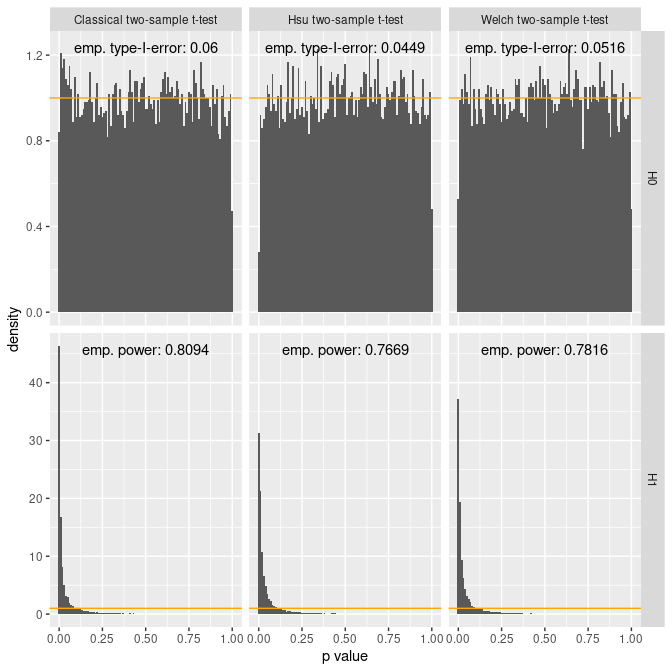qqunif(res.t, alpha = 0.05)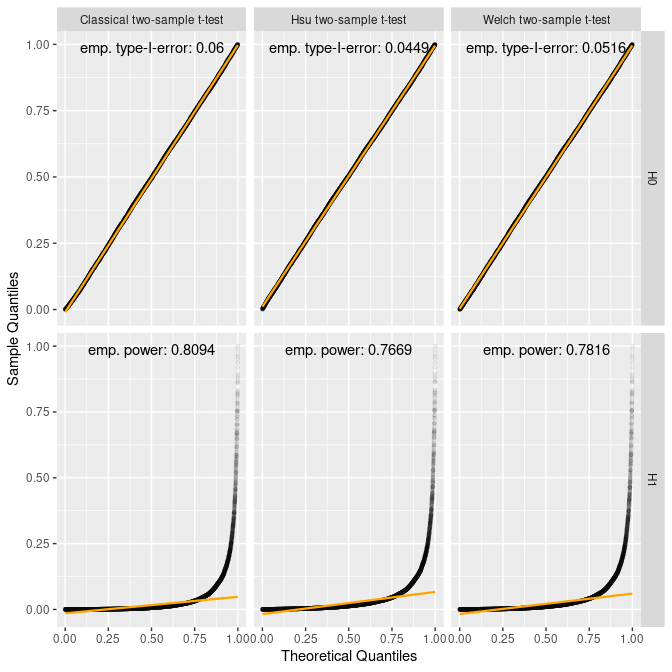volcano(res.t, hex = TRUE)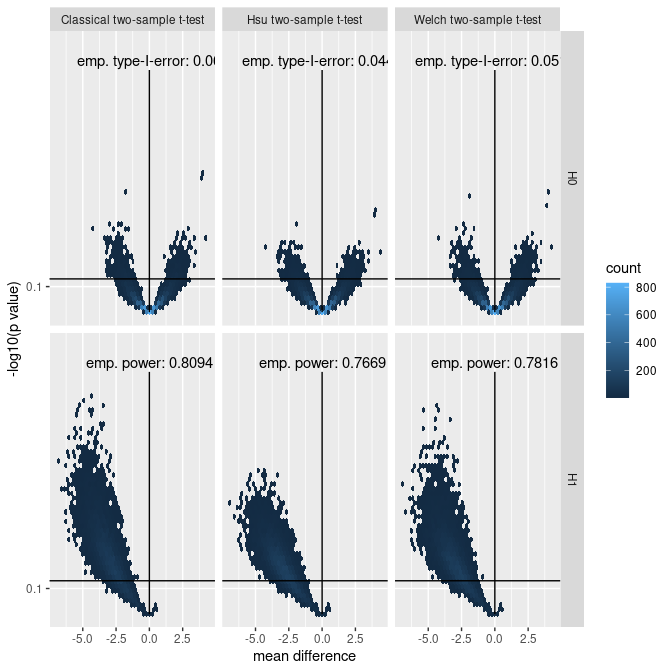##  Wilcoxon-Mann-Whitney tests
hist(res.w)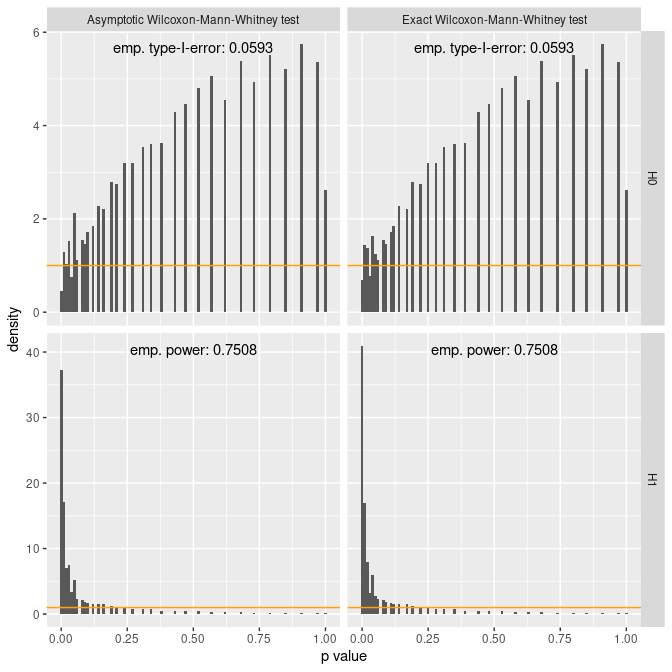qqunif(res.w, alpha = 0.05)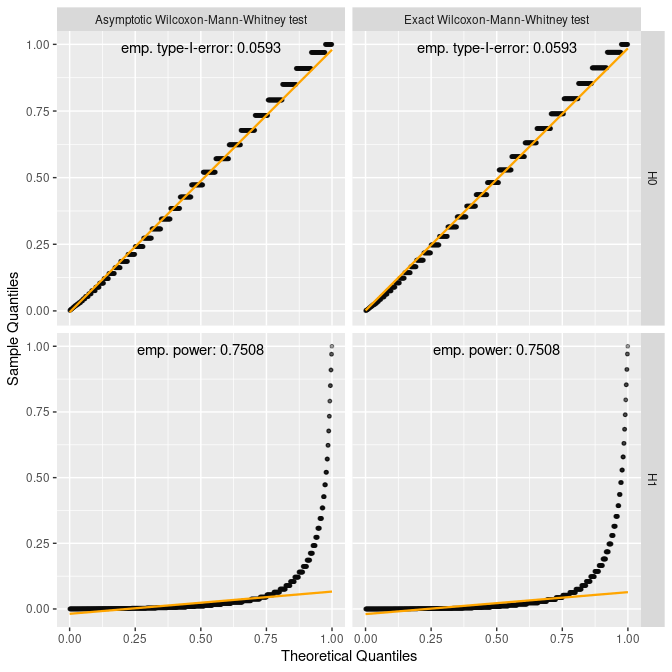We can also generate a volcano plot for the Wilcoxon-Mann-Whitney test, but this would require setting argument conf.int to TRUE, which would lead to a much higher computation time, hence we omitted it here. Furthermore, it is also possible to compute an approximate version of the test by setting argument approximate to TRUE (Hothorn et al. 2008) again by the cost of an increased computation time.

## sessionInfo

sessionInfo()
## R version 4.3.0 Patched (2023-04-21 r84298)
## Platform: x86_64-pc-linux-gnu (64-bit)
## Running under: Linux Mint 21.1
##
## Matrix products: default
## BLAS:   /usr/lib/x86_64-linux-gnu/libf77blas.so.3.10.3
## LAPACK: /usr/lib/x86_64-linux-gnu/atlas/liblapack.so.3.10.3;  LAPACK version 3.10.0
##
## locale:
##   LC_CTYPE=de_DE.UTF-8       LC_NUMERIC=C
##   LC_TIME=de_DE.UTF-8        LC_COLLATE=C
##   LC_MONETARY=de_DE.UTF-8    LC_MESSAGES=de_DE.UTF-8
##   LC_PAPER=de_DE.UTF-8       LC_NAME=C
##  LC_MEASUREMENT=de_DE.UTF-8 LC_IDENTIFICATION=C
##
## time zone: Europe/Berlin
## tzcode source: system (glibc)
##
## attached base packages:
##  stats     graphics  grDevices utils     datasets  methods   base
##
## other attached packages:
##  MKpower_0.7
##
## loaded via a namespace (and not attached):
##   tidyselect_1.2.0        libcoin_1.0-9           dplyr_1.1.2
##   farver_2.1.1            matrixTests_0.2         bitops_1.0-7
##   fastmap_1.1.1           TH.data_1.1-2           pracma_2.4.2
##  MKdescr_0.8             digest_0.6.31           lifecycle_1.0.3
##  arrangements_1.1.9      survival_3.5-5          magrittr_2.0.3
##  compiler_4.3.0          rlang_1.1.0             sass_0.4.5
##  tools_4.3.0             utf8_1.2.3              yaml_2.3.7
##  knitr_1.42              labeling_0.4.2          multcomp_1.4-23
##  memuse_4.2-3            MKinfer_1.1             withr_2.5.0
##  purrr_1.0.1             grid_4.3.0              stats4_4.3.0
##  fansi_1.0.4             opdisDownsampling_0.8.2 caTools_1.18.2
##  colorspace_2.1-0        mice_3.15.0             ggplot2_3.4.2
##  scales_1.2.1            iterators_1.0.14        MASS_7.3-59
##  cli_3.6.1               mvtnorm_1.1-3           rmarkdown_2.21
##  generics_0.1.3          exactRankTests_0.8-35   twosamples_2.0.0
##  robustbase_0.95-1       httr_1.4.5              DBI_1.1.3
##  cachem_1.0.7            modeltools_0.2-23       splines_4.3.0
##  parallel_4.3.0          matrixStats_0.63.0      mitools_2.4
##  vctrs_0.6.2             boot_1.3-28.1           Matrix_1.5-4
##  sandwich_3.0-2          jsonlite_1.8.4          benchmarkme_1.0.8
##  foreach_1.5.2           qqplotr_0.0.6           tidyr_1.3.0
##  jquerylib_0.1.4         hexbin_1.28.3           glue_1.6.2
##  benchmarkmeData_1.0.4   DEoptimR_1.0-12         codetools_0.2-19
##  gtable_0.3.3            gmp_0.7-1               munsell_0.5.0
##  pkgconfig_2.0.3